Updated by class12maths on Sep 09, 2021
REPORTclass12maths
Owner
12 items   2 followers   21 votes   2 views

# Class 12 Maths IIT JEE Mains

Class 12 Maths is a basics for students intended to go for Engineering studies like IIT JEE Mains, other entrances, boards, and higher studies in Mathematics. It covers all the basic to advance concepts, each & important examples and much more.

1

## Exercise 1.1 Relations and Functions Class 12 Maths IIT JEE Mains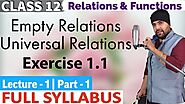Detailed explanation of Empty Relations, Universal Relations and questions related to NCERT Solutions for Exercise 1.1 of Chapter 1 Relations and Functions Class 12 Maths IIT JEE Mains explained by expert teacher.

2

## Exercise 6.2 Application of Derivatives Class 12 Maths IIT JEE Mains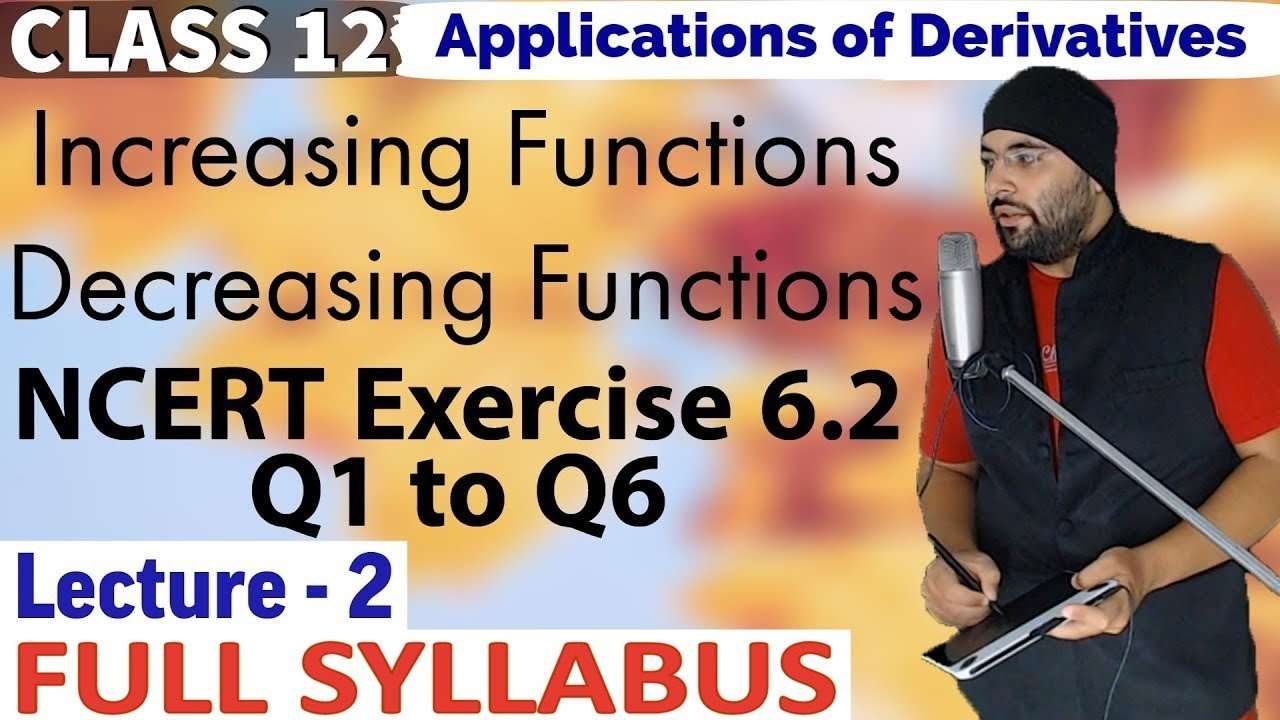Detailed explanation of Increasing Functions, Decreasing Functions and NCERT Solutions for Exercise 6.2 Applications of Derivatives Class 12 Maths IIT JEE Mains explained by expert teacher.

3

## Exercise 5.4 & 5.5 Continuity And Differentiability Class 12 Maths IIT JEE Mains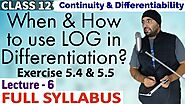Detailed explanation of When & How to use LOG in Differentiation and questions related to NCERT Solutions for Exercise 5.4 and 5.5 Continuity and Differentiability Class 12 Maths IIT JEE Mains explained by expert teacher.

4

## Questions based on Equivalence Relations Chapter 1 Class 12 Maths IIT JEE Mains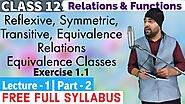Detailed explanation of Reflexive, Symmetric, Transitive, Equivalence Relations and questions based on NCERT Solutions for Exercise 1.1 Relations and Functions Class 12 Maths IIT JEE Mains explained by expert teacher.

5

## Exercise 6.5 Application of Derivatives Class 12 Maths IIT JEE Mains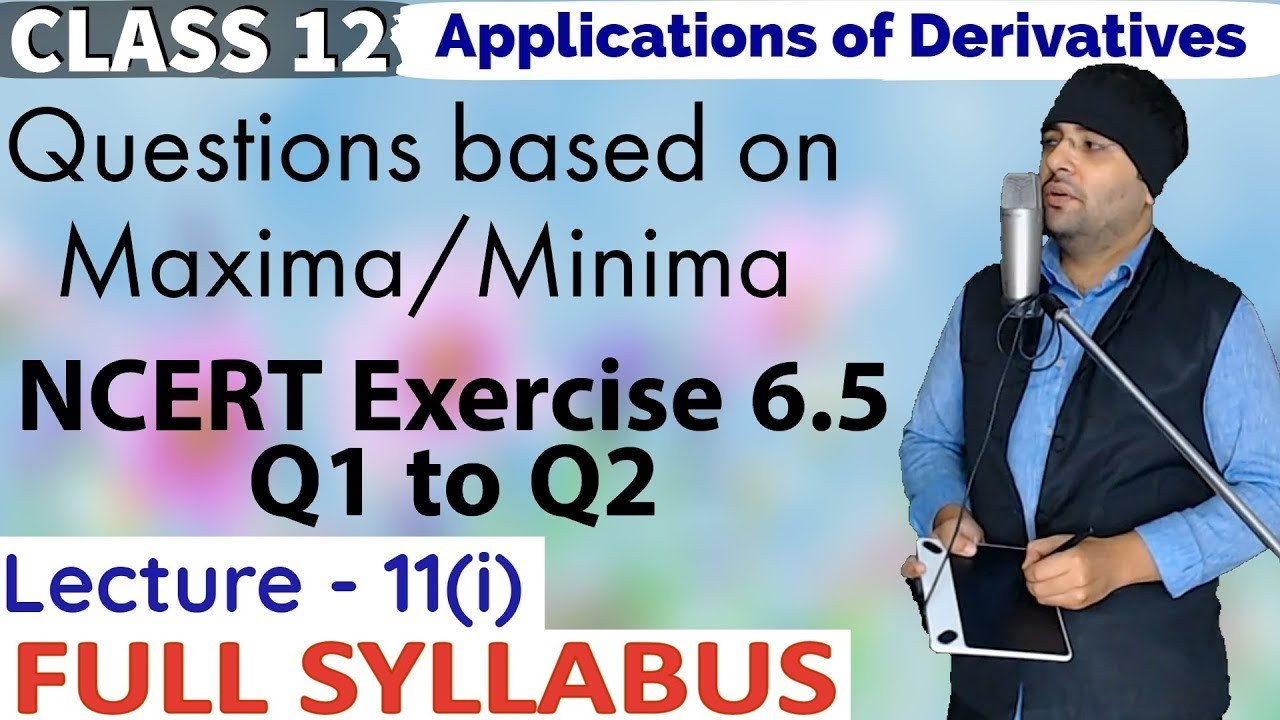Detailed explanation of Questions based on Maxima/ Minima and questions related to NCERT Solutions for Exercise 6.5 Applications of Derivatives Class 12 Maths IIT JEE Mains explained by expert teacher.

6

## Exercise 5.1 Continuity And Differentiability Class 12 Maths IIT JEE Mains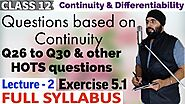Detailed explanation of Questions based on Continuity, other HOTS questions and questions based on NCERT Solutions for Exercise 5.1 Continuity and Differentiability Class 12 Maths IIT JEE Mains explained by expert teacher.

7

## Exercise 10.2 Vector Algebra Class 12 Maths IIT JEE Mains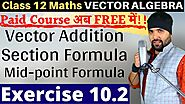Detailed explanation of Vector Addition Triangle Law Parallelogram Law, Section Formula, Mid-Point Formula and questions based on NCERT Solutions of Exercise 10.2 Chapter 10 Vector Algebra Class 12 Maths IIT JEE Mains explained by expert teacher.

8

## NCERT Exercise 4.4 Determinants Class 12 Maths IIT JEE Mains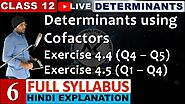Detailed explanation of Determinants using Cofactors and questions based on NCERT Solutions of Exercise 4.4 and 4.5 of Chapter 4 Determinants Class 12 Maths IIT JEE Mains explained by expert teacher.

9

## Three Dimensional Geometry Class 12 Maths IIT JEE Mains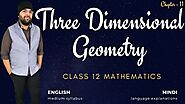Detailed explanation of NCERT Chapter 11 Three Dimensional Geometry Class 12 Maths IIT JEE Mains that will cover basics to advance level concepts, NCERT Solutions, R.D Sharma, R.S Aggarwal and Board’s Question Bank and Important Examples.

10

## Vector Algebra Class 12 Maths Chapter 10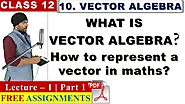Detailed explanation of Vector Algebra, how to represent a vector in mathematics and questions based on NCERT Solutions for Chapter 10 Vector Algebra Class 12 Maths IIT JEE Mains explained by expert teacher.

11

## Exercise 2.2 Inverse Trigonometric Functions Class 12 Maths IIT JEE Mains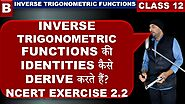Detailed explanation of 'how to drive identities of Inverse Trigonometric Functions' and questions related to NCERT Solutions for Exercise 2.2 Inverse Trigonometric Functions Class 12 Maths IIT JEE Mains explained by expert teacher.

12

## Full syllabus of Class 12 Maths at ₹159 | YouTube Membership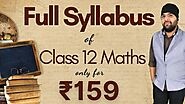High-Quality Video Lectures for Full Syllabus of Class 12 Maths having explanations from basic to advance concepts, each every NCERT Solutions, questions, and important examples, NCERT Exemplar Problem, Board's Question Bank and much more explained by expert teacher at just ₹159.